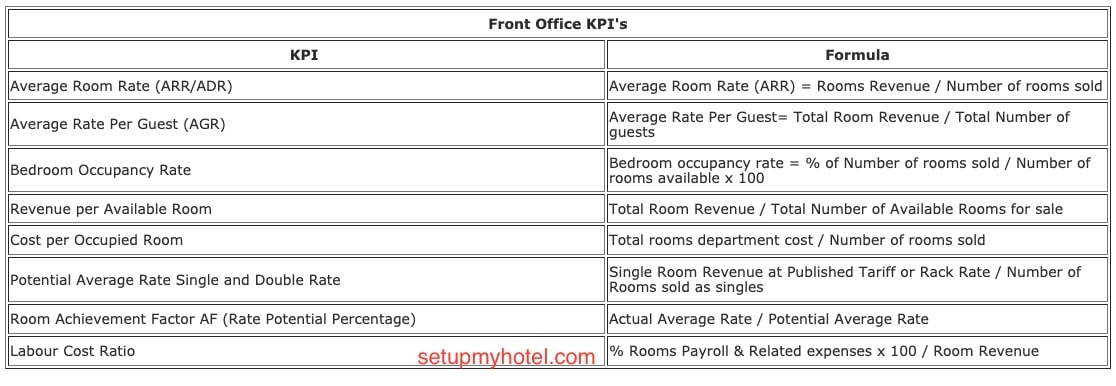# List of KPI (Key Performance Indicators) Used in Hotel Front office

 Front Office KPI's KPI Formula Average Room Rate (ARR/ADR) Average Room Rate (ARR) = Rooms Revenue / Number of rooms sold Average Rate Per Guest (AGR) Average Rate Per Guest= Total Room Revenue / Total Number of guests Bedroom Occupancy Rate Bedroom occupancy rate = % of Number of rooms sold / Number of rooms available x 100 Revenue per Available Room Total Room Revenue / Total Number of Available Rooms for sale Cost per Occupied Room Total rooms department cost / Number of rooms sold Potential Average Rate Single and Double Rate Single Room Revenue at Published Tariff or Rack Rate / Number of Rooms sold as singles Room Achievement Factor AF (Rate Potential Percentage) Actual Average Rate / Potential Average Rate Labour Cost Ratio % Rooms Payroll & Related expenses x 100 / Room Revenue

## 1. Average Room Rate (ARR/ADR):

Although room rates may vary depending on the market segment, many lodging property managers calculate an average room rate (“ARR”). The average room rate reveals the average rate charged per paid room occupied and is calculated by dividing rooms revenue by the number of paid rooms occupied, as follows.

Average Room Rate (ARR or ADR) = Total Rooms Revenue / Number of rooms sold

## 2. Average Rate Per Guest (AGR):

Provides the average revenue contribution by each guest occupied in the hotel, This rate is normally based on every guest in the hotel including children. Some hotels take their AGR without considering children.

Average Rate Per Guest= Total Room Revenue / Total Number of guests

Average Rate Per Guest W/O Child = Total Room Revenue / Total Number of Adults

Online AGR Calculator

## 3. Bedroom Occupancy Rate:

This operating ratio compares the number of rooms sold to the number of rooms available. Managers rely on this ratio in determining whether, or not, the premises are being utilised efficiently and whether expansion is possible.

The bedroom occupancy rate is calculated by dividing the number of rooms sold by the number of rooms available, as follows.

Bedroom occupancy rate = % of Number of rooms sold / Number of rooms available x 100

## 4. Revenue per available room (RevPAR)

This operating ratio compares rooms revenue to the number of rooms available. Revenue per available room (“REVPAR”) is calculated as follows:

Revenue per available room = Total Room Revenue / Total Number of Available Rooms for sale

Online RevPAR Calculator

## 5. Cost Per Occupied Room

This operating ratio compares the cost of the rooms department to the number of rooms sold. Managers rely on this ratio to determine whether, or not, room costs are reasonable. Occupied room cost is calculated by dividing the cost of the rooms department by the number of rooms sold, as follows:

Cost per occupied room = Total rooms department cost / Number of rooms sold

## 6. Potential Average Rate Single and Double Rate:

One of the main computation involved in the front office or revenue management is to calculate the hotel's yield statistics. Potential Average Rate provides the amount of revenue that would have been generated if all the rooms were sold at their published or rack rate.

Potential Average Single Rate = Single Room Revenue at Published Tariff or Rack Rate / Number of Rooms sold as singles

Online Calculator for Potential Average Single & Double Rate

## 7. Room Achievement factor

The Room Achievement Factor is also known as Rate Potential Percentage of a hotel, is defined as the percentage of the Rack Rate that the hotel actually receives by selling their rooms. Room Achievement factor is calculated by dividing the Actual Average Rate (ARR or ADR) by the Potential Average Room Rate.

Additionally, depending upon the hotel's management policy the Actual Average Rate is either divided by the total Rooms Sold or Occupied Rooms. Room Sold = (Occupied Rooms - Complimentary and House use)

Room Achievement Factor = Actual Average Rate / Potential Average Rate

Online Calculator for Room Achievement Factor

## 8. Rooms labour cost

The rooms labour cost percentage is calculated by dividing room payroll and related expenses by total room revenue, as follows:

Rooms labour cost = % Rooms Payroll & Related expenses x 100 / Room Revenue